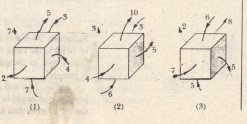# Gauss’ Law

Gauss’ Law

Gauss’ law relates the net flux  of an electric field through a closed surface (a Gaussian surface) to the net charge  that is enclosed by that surface It tells us that By substituting the definition of flux we can also write Gauss’ law as  hold only when the net charge  located in a vacuum or (what is the same for most practical purposes) in air we modify Gauss’ law to include situations in which a material such as mica oil or glass is present In the net charge q enc is the algebraic sum of all the enclosed positive and negative charges and it can be positive negative or zero We include the sign rather than just use the magnitude of the enclosed charge because he sign tells us something about the net flux through the Gaussian surface: If  is positive the net flux is outward  negative the net flux is inward Charge outside the surface no matter how large or how close it may be is not
included in the term in Gauss’ law The exact form or location of the charges
inside the Gaussian surface is also of no concern the only things that matter on the right side of  are the magnitude and sign of the net enclosed charge.
The quantity E on the left side of  however is the electric field resulting
from all charges both those inside and those outside the Gaussian surface This may seem to be inconsistent but keep in mind what we saw in Sample Problem contributes zero net flux through the surface because as many field lines due to that charge enter the surface as leave it Let us apply these ideas to which shows two point charges, equal in magnitude but opposite in sign and the field lines describing the electric fields that they set up in the surrounding space Four Gaussian surfaces are also shown in cross section Let us consider each in turn.Surface SI’ The electric field is outward for all points On this surface Thus the flux of the electric field through this surface is positive and so’ is the net charge within the surface. as Gauss’  requires (That is s positive must be also S2′ The electric field is inward for all points on this surface Thus, the flux of the electric field is negative and so is the enclosed charge\as Gauss’ law requires Surface S3′ This surface encloses no charge and thus qenc = Q Gauss’ law   requires that the net flux of the electric field through this surface be zero That is reasonable because all the field lines pass entirely through the surface entering it at the top and leaving at the bottom Surface S4′ This surface encloses no net charge, because the enclosed positive arid negative charges have equal magnitudes Gauss’ law requires that the net flux of the electric field through this surface be zero That is reasonable because there are as many field lines leaving surface S4 as entering it What would happen if we were to bring an enormous charge Q up close to surface S4 in  The pattern of the field lines would certainly change. but the net flux for each of the four Gaussian surfaces would not change We can understand this because the field lines associated with the added Q would pass entirely through each of the four Gaussian surfaces making no contribution to the net flux through any of them The value of Q would not enter Gauss’ law in any way because Q lies outside all four of the Gaussian surfaces that we are considering   The figure shows three situations in which a Gaussian cube sits in an electric field The arrows and the values indicate the directions of the field lines and the magnitudes (in N  m /C) of the flux through the six sides of each cube (The lighter arrows are for the hidden faces\) In which situations does the cube enclose (a) positive net charge (b) a negative net charge and (c) zero net charge.